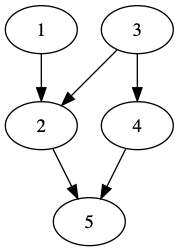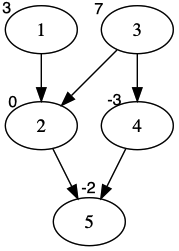# 模型描述

## 最大权闭合子图

### 闭合子图### 最大权闭合子图# 代码

（以链接里的题目代码为准，大同小异）

#include <algorithm>
#include <iostream>
#include <cstring>
#include <climits>
#include <cstdio>
#include <vector>
#include <cstdlib>
#include <ctime>
#include <cmath>
#include <queue>
#include <stack>
#include <map>
#include <set>

#define Re register
#define LL long long
#define U unsigned
#define FOR(i,a,b) for(Re int i = a;i <= b;++i)
#define RFOR(i,a,b) for(Re int i = a;i >= b;--i)
#define SFOR(i,a,b,c) for(Re int i = a;i <= b;i+=c)
#define CLR(i,a) memset(i,a,sizeof(i))
#define BR printf("--------------------\n")
#define DEBUG(x) std::cerr << #x << '=' << x << std::endl

const int MAXN = 2000+5;

struct Node{
struct Edge *first,*cur;
int level;
}node[MAXN];

struct Edge{
Node *s,*t;
Edge *next,*rev;
int cap,flow;
}pool,*frog = pool;

Edge *New(Node *s,Node *t,int cap){
Edge *ret = ++frog;
*ret = (Edge){s,t,s->first,NULL,cap,0};
return ret;
}

inline void add(int u,int v,int cap,int rev=0){
node[u].first = New(&node[u],&node[v],cap);
node[v].first = New(&node[v],&node[u],rev);
node[u].first->rev = node[v].first;
node[v].first->rev = node[u].first;
}

int N,S,T;

bool bfs(Node *s,Node *t){
FOR(i,0,N){
node[i].cur = node[i].first;
node[i].level = 0;
}
std::queue<Node *> q;
s->level = 1;q.push(s);
while(!q.empty()){
Node *v = q.front();q.pop();
for(Edge *e = v->first;e;e = e->next){
if(e->flow < e->cap && !e->t->level){
e->t->level = v->level + 1;
if(e->t == t) return true;
q.push(e->t);
}
}
}
return false;
}

int dfs(Node *v,Node *t,int limit = INT_MAX){
if(v == t) return limit;
int flow;
for(Edge *&e = v->cur;e;e = e->next){
if(e->flow < e->cap && e->t->level == v->level + 1){
if((flow = dfs(e->t,t,std::min(limit,e->cap-e->flow)))){
e->flow += flow;
e->rev->flow -= flow;
return flow;
}
}
}
return 0;
}

int dinic(){
int ans=0,flow;
while(bfs(&node[S],&node[T])){
while((flow = dfs(&node[S],&node[T]))){
ans += flow;
}
}
return ans;
}

int n,m,sum;

int main(){
scanf("%d%d",&n,&m);
N = n+m+1;
S = 0,T = N;
FOR(i,1,m){
int x;scanf("%d",&x);
}
FOR(i,1,n){
int a,k;
scanf("%d%d",&a,&k);
}